Recent News

# Anomaly Detection Using the Bag-of-Words ModelI am going to show in detail one use case of unsupervised learning: behavioral-based anomaly detection. Imagine you are collecting daily activity from people. In this example, there are six people (S1-S6). When all the data are sorted and pre-processed, the result may look like this list:

• S1 = eat, read book, ride bicycle, eat, play computer games, write homework, read book, eat, brush teeth, sleep
• S2 = read book, eat, walk, eat, play tennis, go shopping, eat snack, write homework, eat, brush teeth, sleep
• S3 = wake up, walk, eat, sleep, read book, eat, write homework, wash bicycle, eat, listen music, brush teeth, sleep
• S4 = eat, ride bicycle, read book, eat, play piano, write homework, eat, exercise, sleep
• S5 = wake up, eat, walk, read book, eat, write homework, watch television, eat, dance, brush teeth, sleep
• S6 = eat, hang out, date girl, skating, use mother’s CC, steal clothes, talk, cheating on taxes, fighting, sleep

S1 is the set of the daily activity of the first person, S2 of the second, and so on. If you look at this list, then you can pretty easily recognize that activity of S6 is somehow different from the others. That’s because there are only six people. What if there were six thousand? Or six million? Unfortunately, there is no way you could recognize the anomalies. But machines can. Once a machine can solve a problem on a small scale, it can usually handle the large scale relatively easily. Therefore, the goal here is to build an unsupervised learning model that will identify S6 as an anomaly.

What is this good for? Let me give you two examples.

The first example is traditional audit log analysis for the purpose of suspicious activity detection. Let’s consider e-mail. Almost everyone has their own daily usage pattern. If this pattern suddenly changes, this is considered suspicious. It might mean that someone has stolen your credentials. It might also mean that you just changed your habits. Machines can’t know the underlying reason. But they can analyze millions of accounts and pick up only the suspicious ones, which is typically a very small number. Then, the operator can manually call to these people and discover what is going on.

For the second example, imagine you are doing pre-sales research. You employ an agency to make a country-wide survey. There is a question like, “Please give us 40-50 words of feedback.” Let’s say you get 30,000 responses that satisfy the length requirement. Now, you want to choose the responses that are somehow special — they might be extremely good, extremely bad, or just interesting. All of these give you valuable insight and possible direction for the future. Since the overall amount is relatively high, any human would certainly fail in this job. But for machines, this is a piece of cake.

Let’s look at how to teach the machine to do the job.

The example project is available on the original post. It is a Java Maven project. Unpack it into any folder, compile by `mvn package`, and run by executing `java -jar target/anomalybagofwords-1.0.jar 0.5 sample-data-small.txt`. If you run the program this way, it will execute the described process over the cooked dataset and identifies S6 as an anomaly. If you want to drill down the code, then start with `BagofwordsAnomalyDetectorApp`  class.

## Terminology

Let’s briefly establish useful terminology.

Bag of words is a set of unique words within a text in which each word is paired with the number of its occurrence. One specific point is that the order of words is ignored by this structure. If a word is not presented in the text, then its occurrence will be considered to be 0. For example, a bag of words for eat, read book, ride bicycle, eat, play computer games, write homework, read book, eat, brush teeth, sleep can be written as the following table:

Word Number of occurrences
eat 3
book 2
ride 1
bicycle 1
play 1
computer 1
games 1
write 1
homework 1
brush 1
teeth 1
sleep 1

Sometimes, you can find the visualization as a histogram: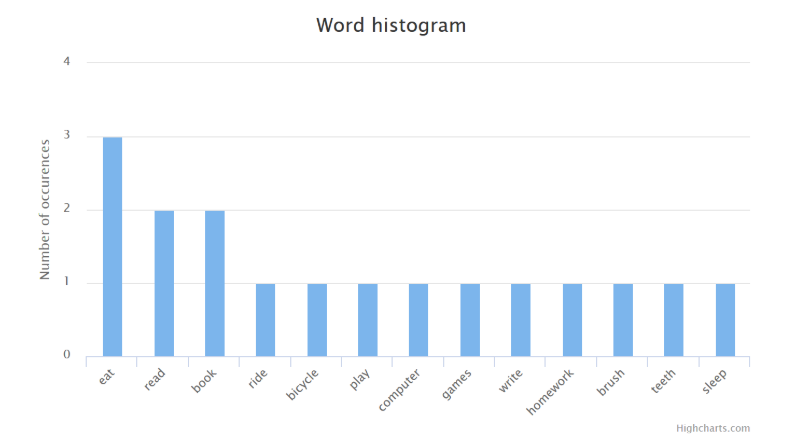The notation B(x) will be used for the bag of words. Following is the example for S1: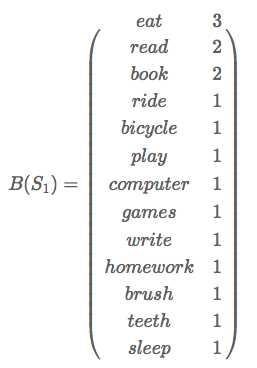The next term involves the distance between two bags of words. The distance will be written as |B(x)−B(y)| and is calculated as a sum of absolute values of the differences for all words appearing in both bags. Following is the example.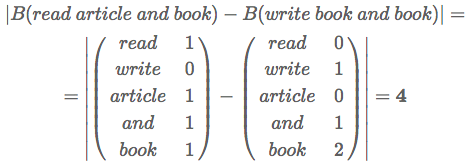Applying this definition, you can calculate the distance between all the example sequences; for example, |B(S1)−B(S2)|=12 and |B(S1)−B(S6)|=30. The latter is higher because S1 and S6 are more different in words than S1 and S2. This is an analogy to the distance between two points in the space.

Last term is probability density function. Probability density function is a continuous function defined over the whole real numbers space, which is greater or equal to zero for every input and integral over the whole space. Notation P(x) will be used. More formally, this means the following: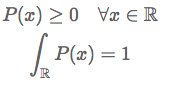A typical example of probability density function is normal distribution. The example source code uses a more complex one called normal distribution mixture. Parameter x is called random variable. In a very simplistic way, the higher P(x) is, the more “likely” variable x is. If P(x) is low, then the variable x is falling away from the standard. This will be used when setting up the threshold value. Finally, let’s make a note about how to create a probability density from a finite number of random variables. If [x1,…,xN] is the set of N random variables (or samples you can collect), then there is a process called estimation which transforms this finite set of numbers into a continuous probability density function P. An explanation of this process is out of scope for this article; just remember there is such a thing. In particular, the following example uses a variation of the EM algorithm: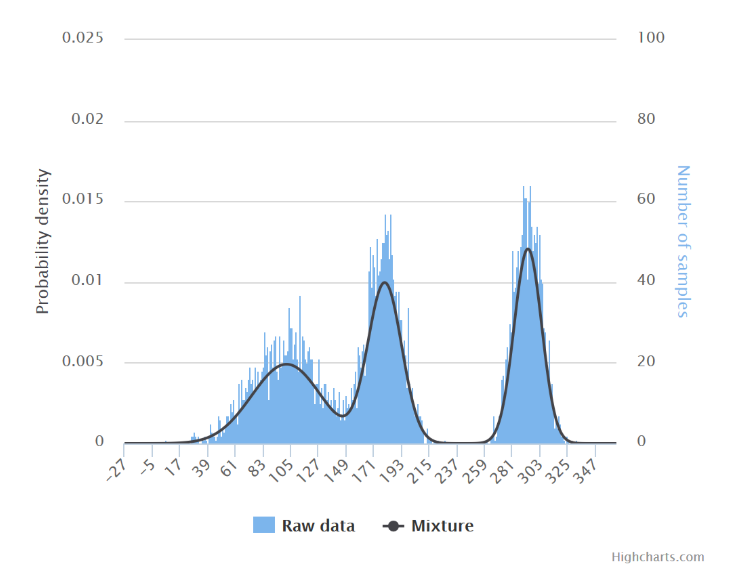Normal distribution mixture estimated from 5,000 samples.

## Process

Now, it’s time to explain the process. The whole process can be separated into two phases: training and prediction. Training is the phase when all the data is iterated through and a relatively small model is produced. This is usually the most time-consuming operation. The outcome is sometimes called a predictive model. Once the model is prepared, the prediction phase comes into place. In this phase, an unknown data record is examined by the model. Next, let’s drill down the details.

### Training Phase

There are required two inputs for the training phase.

1. Set of activities [S1,…,SN]. This might be the example set from the beginning.
2. Sensitivity factor α, which is just the number initially picked up by a human that α≥0. More on this one later.

The whole process is pretty straightforward and you can find the implementation in the source code — class `BagofwordsAnomalyDetector` and method `performTraining`.

1. For each activity, calculate a bag of words. The result of this step is N bags of words [B(S1),…,B(SN)].
2. Calculate random variables. One random variable is calculated for each bag of words. Result of this step is N random variables [x1,…,xN]. The formula for calculation is: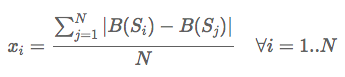3. Estimate the probability density of function PP. This process takes random variables [x1,…,xN] and produces probability density function PP. A variation of the EM algorithm is used in the example program.
4. Calculate the threshold value θ. This value is calculated according to the following formula:The higher α is, the more activities will be identified as anomalies. The problem with the unsupervised learning model is that data is not labeled and therefore there is no way to know what the correct answers are and how to set up the optimal α. Therefore, some rules of thumb are used instead. For example, set up α to report a reasonable percentage of activity as an anomaly. Typically, it is required that the amount of identified anomalies must be manageable by the human investigators. In the bigger system, there is usually a feedback loop that incrementally adjusts α until the optimal value is reached. This is then called reinforcement learning. For this small example, α was picked up manually as 0.5 by trial-and-error just to reach the goal.
5. Store all bags of words P and θ for later usage.

When training phase finishes, the model is ready to be used in the prediction phase.

### Prediction Phase

This is the phase when potentially unseen activities are tested by the model. The model then evaluates them and says whether the activities are considered to be anomalies.

The whole process works for each activity SU separately (U stands for “unknown”). This can be summarized by:

1. Calculate bag of words B(SU).
2. Calculate random variable xU as: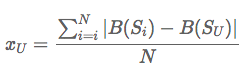3. If P(xU)≤θ, then activity SU is considered to be an anomaly. Otherwise, the activity is considered to be normal.

## Summary

You have learned about a relatively simple model for identifying unusual sequences from a bulk of data. Now, you can play with source code, try different variations, and see how this affect the result. Here are a few ideas to start with.

• Normalize bags of words. In other words don’t count the absolute number, just relative frequency.
• Use chunks of more than one word. This is then called the n-gram model.
• Try to implement different ways of measuring the distance between items, for example, sequence alignment.

### Key Takeaways

• There is no knowledge about what the correct outcome is at the beginning of the unsupervised learning. Therefore, best guesses and feedback loops are implemented.
• Predictive models are usually built in the training phase and then used to classify unknown data in the prediction phase.
• In order to be able to find the outliers, abstract features like sentences or actions need to be transformed into a measurable form. After that, probability and statistics are used to establish baselines and find outliers.

This UrIoTNews article is syndicated fromDzone